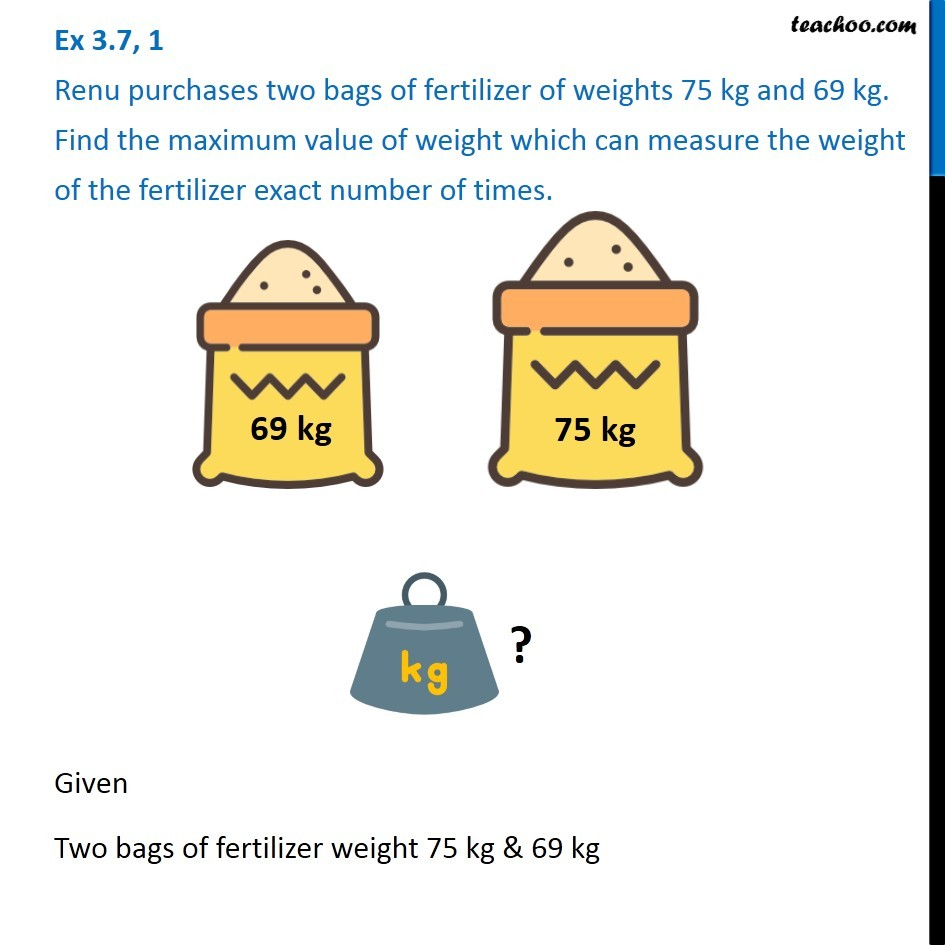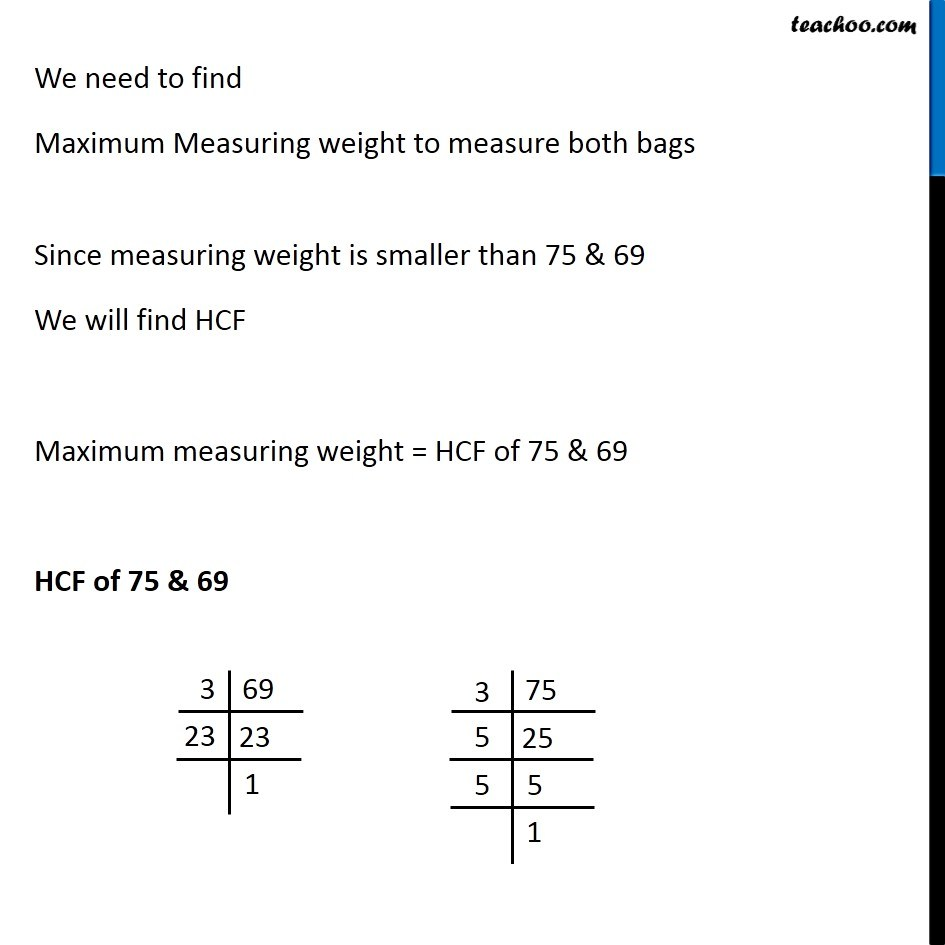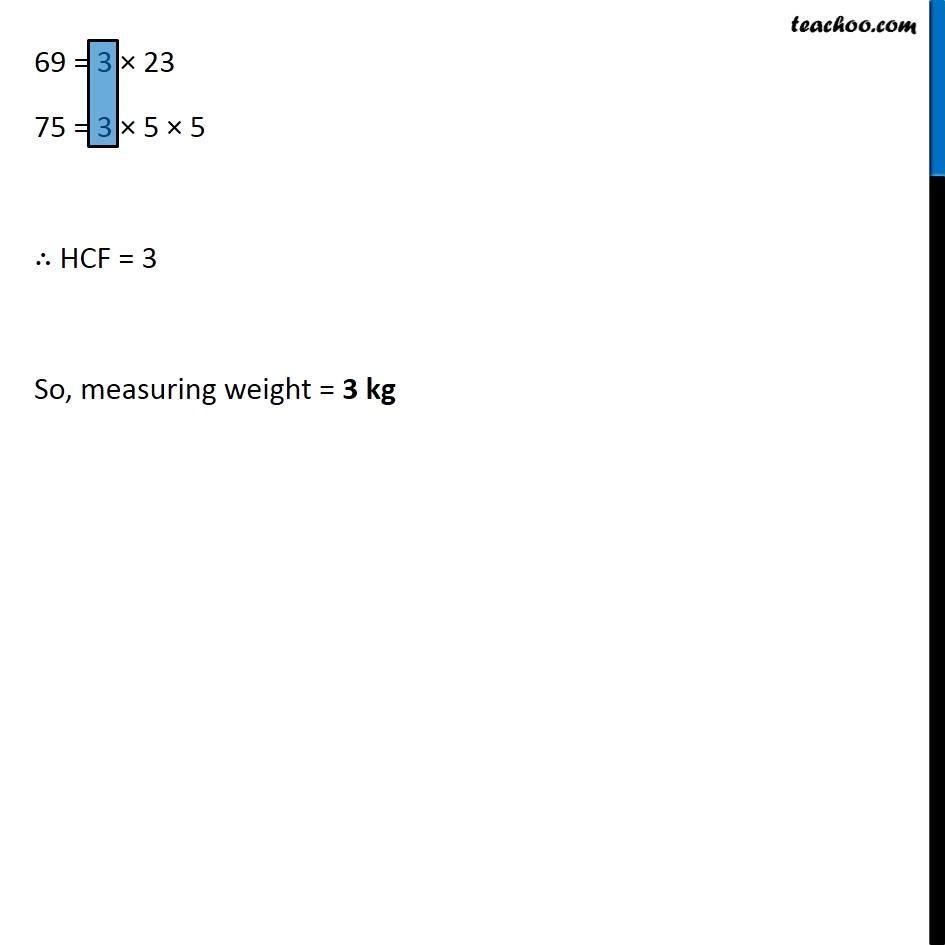1. Chapter 3 Class 6 Playing with Numbers
2. Concept wise
3. Statement questions on HCF

Transcript

Given Two bags of fertilizer weight 75 kg & 69 kg We need to find Maximum Measuring weight to measure both bags Since measuring weight is smaller than 75 & 69 We will find HCF Maximum measuring weight = HCF of 75 & 69 HCF of 75 & 69 69 = 3 × 23 75 = 3 × 5 × 5 ∴ HCF = 3 So, measuring weight = 3 kg

Statement questions on HCF